# 2nd PUC Accountancy Question Bank Chapter 5 Accounting Ratios

## Karnataka 2nd PUC Accountancy Question Bank Chapter 5 Accounting Ratios

### 2nd PUC Accountancy Accounting Ratios NCERT Textbook Questions and Answers

Percentage off calculator tool calculates percent off , increase and decrease with percentage discount formula.

Question 1.
What do you mean by Ratio Analysis?
Ratio Analysis is a technique of financial analysis. It describes the relationship between various items of Balance Sheet and Income Statements. It helps us in ascertaining profitability, operational efficiency, solvency, etc. of a firm. It may be expressed as a fraction, proportion, percentage and in times. It enables budgetary controls by assessing qualitative relationship among different financial variables.

Ratio Analysis provides vital information to various accounting users regarding the financial position and viability and performance of a firm. It also lays down the basic framework for decision making and policy designing by management.

Question 2.
What are the various types of ratios?
Accounting ratios are classified in the following two ways.
1. Traditional Classification: This classification is based on the financial statements, i.e. profit and Loss Account and Balance Sheet. The Traditional Classification further bifurcates accounting ratios as:
(a) Income Statement Ratios: These are those ratios whose all the elements belong only to the Trading and Profit and Loss Account, like Gross Profit Ratio, etc.
(b) Balance Sheet Ratios: These are those ratios whose all the elements belong only to the Balance Sheet, like Current Ratio, Debt Equity Ratio, etc,
(c) Composite Ratios: These are those ratios whose elements belong both to the Trading and Profit and Loss Account as well as to the Balance Sheet, like Debtors Turnover Ratio, etc.

2. Functional Classification: This classification reflects the functional need and the purpose of calculating ratio. The basic rationale to compute ratio is to ascertain liquidity, solvency, financial performance and profitability of a business. Consequently, the Functional Classification classifies various accounting ratios as:
(a) Liquidity Ratio: These ratios are calculated to determine short term solvency.
(b) Solvency Ratio: These ratios are calculated to determine long term solvency.
(c) Activity Ratio: These ratios are calculated for measuring the operational efficiency and efficacy of the operations. These ratios relate to sales or cost of goods sold.
(d) Profitability Ratio: These ratios are calculated to assess the financial performance and the financial viability of the business.Question 3.
What relationships will be established to study?
(a) Inventory Turnover
(b) color Turnover
(c) Payables Turnover
(d) king Capital Turnover
(a) Inventory Turnover Ratio: This ratio is cc puted to determine the efficiency with which the stock is used. This ratio is b; scd on the relationship between cost of goods sold and average stock kept during the year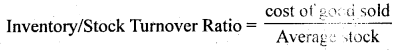Cost of goods sold = Opening Stock + Purchases + Direct expenses – Closing Stock
Or cos to goods sold = Net sales – Gross Profit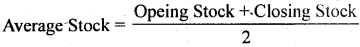(b) Debtors Turnover Ratio: This ratio is computed to determine the rate at which the amount is collected from the debtors. It establishes the relationship between net credit sales and average accounts receivables,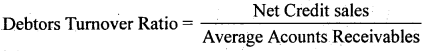Net Credit Sales = Total Sales – Cash Sales
Average Account Receivables =(c) Payable Turnover Ratio: This ratio is known as Creditors Turnover Ratio.’ It is computed to determine the rate at which the amount is paid to the creditors. It establishes the relationship between net credit purchases and average accounts payables.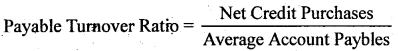Net Credit purchases = Total Purchases – Cash Purchases
Average Account Payable(d) Working Capital Turnover Ratio: This ratio is computed to determine how efficiently the working capital is utilised in making sales. It establishes the relationship between net sales and working capital.
Working Capital Turnover Ratio =Net Sales = total Sales – Sales Return
Working Capital = Current Assets – Current Liabilities.

Question 4.
Why would the inventory turnover ratio be more important when analysing a grocery store than an insurance company?
Grocery store is a trading concern and involved in business of buying and selling of grocery. It keeps stock of various groceries to meet the requirement of the customers and it should calculate the inventory turnover ratio. Hence, this ratio is more important for a grocery store then it is for an insurance company as the latter does not need to maintain any stock of goods sold. The insurance company is engaged in delivering service that is intangible and, thus, cannot be stored.

Question 5.
The liquidity of a business firm is measured by its ability to satisfy its long- term obligations as they become due? Comment.
The liquidity of a business firm is measured by its ability to pay its long term obligations, Die long term obligations include payments of principal amount on the due date and payments of interests on the regular basis. Long term solvency of any business can be calculated on the basis of the following ratios:

Debt-Equity Ratio: It depicts the relationship between the borrowed fund and owner’s funds. The lower the debt-equity ratio higher will be the degree of security to the lenders. A low debt-equity ratio implies that the company can easily meet its’long term obligations.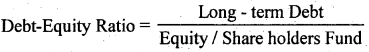Total Assets to Debt Ratio: It shows the relationship between the total assets and the long term loans A high Total Assets to Debt Ratio implies that more assets are financed by the owner’s fund and the company can easily meet its long-term obligations.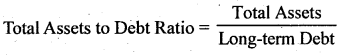Interest Coverage Ratio: This ratio depicts the relationship between amount of profit utilise for paying interest and amount of interest payable. A high Interest Coverage Ratio implies that the company can easily meet all its interest obligations out of its profit.Proprietary Ratio: It shows the relationship between the Shareholders Fund and the Total Assets. This ratio reveals the financial position of a business. The higher the ratio the higher will be the degree of safety for.the creditors. It is calculated as: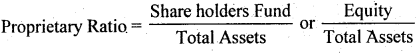Question 6.
The average age of inventory is viewed as the average length of time inventory, is held by the firm or as the average number of day’s sales in inventory. Explain.
Inventory Turnover Ratio: This ratio is computed to determine the efficiency with which the-stock is used. This ratio is based on the relationship between cost of goods sold and rage stock kept during the year. It shows the rate with which the stock is turned into sales Lite number of times the stock in turned into sales during the year. In other words, this ratio reveals the average length of time for which the inventory is held by the firm.Cost of Goods Sold = Opening Stock + Purchases + Direct Expenses – Closing Stock
or, Cost of goods sold = Net Sales – Gross Profit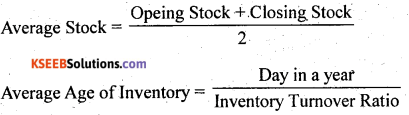Question 1.
Who are the users of financial ratio analysis? Explain the significance of ratio analysis to them?
The users of financial ratio analysis are as follows:
1. Investors
2. Management
3. Short term Creditors
4. Long term Creditors.
The following points signify the importance of ratio analysis for these users.

1. Investors: The main concern for the investors is the security of the funds invested by them in the business and returns on their investments. The security of the funds is directly. related to the profitability and operational efficiency of the business. Consequently, they are interested in knowing Earnings per Share, Return on Investment and Return on Equity.

2. Management: They uses ratio analysis to determine how effectively the assets are being used. They are interested in future growth and prospects. They design various policy measures and draff future plAnswer: Consequently, they are interested in Activity Ratios and Profitability Ratios like, Net Profit Ratio, Debtors Tumover Ratio, Fixed Assets Tumover Ratios, etc.

3. Short-term Creditors: Short-term creditors are interested in timely payment of their debts in short run. Consequently, they are interested in Liquidity Ratios like, Current Ratio, Quick Ratios etc. These ratios reveal the current financial position of the business.

4. Long-term Creditors: Long-term creditors provide funds for more than one year, so they are interested in long term solvency of the firm and in assessing the ability of the firm to pay timely interests. Consequently, they are interested in calculating Solvency Ratios like,. Debt-Equity Ratio, Proprietary Ratio, Total Assets to Debt Ratio, Interest Coverage Ratio, etc.

Question 2.
What are liquidity ratios? Discuss the importance of current and liquid ratio.
Liquidity ratios are calculated to determine the short-term solvency of a business, i.e. the ability of the business to pay back its current dues. Liquidity means easy conversion of assets into cash without any significant loss and delay. Short-term creditors are interested in ascertaining liquidity ratios for timely payment of their debts. Liquidity ratio includes:

Current Ratio: It explains the relationship between current assets and current liabilities. It is calculated as: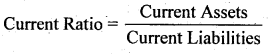Liquid Ratio or Quick Ratio: It explains the relationship between liquid assets and current liabilities. It indicates whether a firm has sufficient funds to pay its current liabilities immediately. It is calculated as:Liquids Assets = Current Assets – Stock – Prepaid ExpensesQuestion 3.
How would you study the solvency position of the firm?
Solvency position of a firm is studied with the help of the Solvency Ratios. Solvency ratios are the measures of the long-term financial position of the firm in terms of its ability to pay its long-term liabilities. In other words, the solvency of the firm is measured by its ability to pay. its long term obligation on the due date. Long term solvency of any business can be calculated on the basis of the following ratios:

Debt-Equity Ratio: It depicts the relationship between the borrowed fund and owner’s funds. The lower the debt-equity ratio higher will be the degree of security to the lenders. A low debt-equity ratio implies that the company can easily meet its long term obligations. Equity or the Shareholders Fund includes.
Preference Share Capital, Equity Share Capital, Capital Reserve, Securities
Premium, General Reserve less Accumulated Loss and Fictitious AssetsTotal Assets to Debt Ratio: It shows the relationship between the total assets and the long term loans A high Total Assets to Debt Ratio implies that more assets are financed by the owner’s fund and the company can easily meet its long-term obligations. Total Assets includes all fixed and current assets except fictitious assets like, Preliminary Expenses, Underwriting Commission, etc. Debt includes all long-term loans that are to be repaid after one year. It includes debentures, mortgage loans, bank loans, loans from other financial institutions, etc.Interest Coverage Ratio: This ratio depicts the relationship between amount of profit utilise for paying interest and amount of interest payable. A high Interest Coverage Ratio implies that the company can easily meet all its interest obligations out of it’s profit.Proprietary Ratio: It shows the relationship between the Shareholders Fund and the Total Assets. This ratio reveals the financial position of a business. The higher the ratio the higher will be the degree of safety for the creditors, It is calculated as:
Proprietary Ratio

Question 4.
What are important profitability ratios? How are they worked out?
Profitability ratios are calculated on the basis of profit earned by a business. This ratio gives a percentage measure to assess the financial viability, profitability and operational efficiency of the business. The various important Profitability Ratios are as follows:
Gross Profit Ratio: It shows the relationship between Gross Profit and Net Sales. It depicts the trading efficiency of a business. A higher Gross Profit Ratio implies a better position of a business, whereas a low Gross Profit Ratio implies an inefficient unfavourable sales policy.Gross Profit = Net Sales – Cost of Goods Sold
Net sales = Total Sales – Sales Return
Cost of Goods Sold = Opening Stock + Purchases + Direct Expenses — Closing Stock

Operating Ratio: It shows the relationship between Cost of Operation and Net Sales. This ratio depicts the operational efficiency of a business. A low Operating Ratio implies higher operational efficiency of the business. A low Operating Ratio is considered better for the business as it enables the business to be left with a greater amount after covering its operation costs to pay for interests and dividends.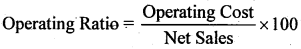Operating Cost = Cost of Goods Sold + Operating Expenses
Cost of Goods Sold = Sales – Gross Profit

Operating Profit Ratio: It shows the relationship between the Operating Profit and Net Sales. It helps in assessing the operational efficiency and the performance of the business.Operating Profit Ratio = 100 – Operating Ratio
Operating Profit = Sales – Operation cost

Net Profit Ratio: It shows the relationship between net profit and sales. Higher ratio is better for firm. It depicts the overall efficiency of a business and acts as an important tool to the investors for analysing and measuring the viability and performance of the business.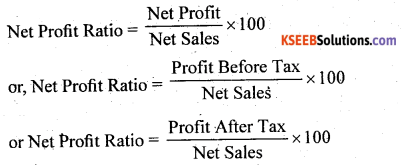Net Sales = Total Sales – Sales Return

Return on Investment or Capital Employed: It shows the relationship between the profit earned and the capital employed to earn that profit. It is calculated as:Capital Employed = Fixed Assets + Current Assets- Current Liabilities
Or, Capital Employed = Share Capital + Reserve and Surplus + Long-term Funds-Fictitious Assets

Earning per Shares: It shows the relationship between the amount of profit available to distribute as dividend among the equity shareholders and number of equity shares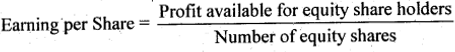Profit available for equity shareholers = Net Profit after tax

Dividend payout Ratio: It shows the relationship between the dividend per share and earnings per share. This ratio depicts the amount of earnings that is distributed in the form of dividend among the shareholders. A high Dividend Payout Ratio implies a better position and goodwill of the business for the shareholders.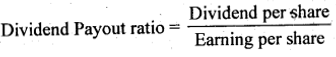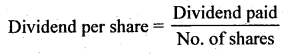Price Earnings Ratio: It shows the relationship between the market price of a share and the earnings per share. This ratio is the most common tool that is used in the stock markets. This ratio depicts the degree of reliance and trust that the shareholders have on the business.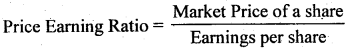Question 5.
Financial ratio analysis is conducted by four groups of analysts: managers, equity investors, long-term creditors, and short-term creditors. What is the primary emphasis of each of these groups in evaluating ratios?
Financial ratio analysis is conducted by four groups of analysts: managers, equity investors, long term creditors and short term creditors. The primary emphasis of each of these groups in evaluating ratios is:
1. Management: They use ratio analysis to determine how effectively the assets are being used. They are interested in future growth and, prospects. They design various policy measures and draft future plans Consequently, they are interested in Activity Ratios and Profitability Ratios like Net Profit Ratio, Debtors Turnover Ratio, Fixed Assets Turnover Ratios, etc..

2. Equity Investors: The main concern for the investors is the security of the funds invested by them in the business and returns on their investments. The security of the funds is directly related to the profitability and operational efficiency of the business. Consequently, they are interested in knowing Earnings per Share, Return on Investment and Return on Equity.

3. Long-term Creditors: Long-term creditors provide funds for more than one year, so they are interested in long term solvency of the firm and in assessing the ability of the firm to pay timely interests. Consequently, they are interested in calculating Solvency Ratios like, Debt-Equity Ratio, Proprietary Ratio, Total Assets to Debt Ratio, Interest Coverage Ratio, etc.

4. Short-term Creditors: Short-term creditors are interested in timely payment of their debts in short run. Consequently, they are interested in Liquidity Ratios like, Current Ratio, Quick Ratios etc. These ratios reveal the current financial position of the business.

Question 6.
The current ratio provides a better measure of overall liquidity only when a firm’s inventory cannot easily be converted into cash. If inventory is liquid, the quick ratio is a preferred measure of overall liquidity. Explain.
Current Ratio: It explains the relationship between current assets and current liabilities. It is calculated as:Currents Assets are those assets that are easily converted into cash within a short period of time like cash in hand, cash at bank, marketable securities, debtors, stock, bills receivables, prepaid expenses, etc.

Current Liabilities are those liabilities that are to be repaid within a year like bank overdraft, bills payables, Short-term creditors, provision for tax, outstanding expenses etc:

Liquid Ratio: It explains the relationship between liquid assets and current liabilities. It indicates whether a firm has sufficient funds to pay its current liabilities immediately. It is calculated as: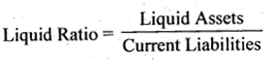Liquid Assets = Current Assets – Stock – Prepaid Expenses

Generally, Current Ratio is preferable for such type of business where the stock or the inventories cannot easily be converted into cash like heavy machinery manufacturing companies, locomotive companies, etc. This is because, the heavy stocks like machinery, Heavy tools etc. cannot be easily sold off. But on the other hand, the businesses where the stock can be easily realised or sold off regard Liquid Ratio to be more suitable measure to reveal their liquidity position. For example, the inventories of a service sector company are very liquid as there is no stock kept for sale, so they prefer Liquid Ratio as a measure of overall liquidity.

Moreover, sometimes companies prefer to resort to Liquid Ratio instead of Current Ratio, if the prices of the stock held are prone to fluctuate. This is because if the prices of the inventories fluctuate more, then this may affect their liquidity position of the business and may reduce (or overcast) the Current Ratio. Consequently, they prefer Liquid Ratio as it excludes inventories and stocks.

Thirdly, if the stock forms the major portion of a company’s current assets, then they would prefer Current Ratio and not Liquid Ratio. This is because their current assets mostly consist of stock. The Liquid Ratio of such company will be very low as liquid assets exclude stock. This will reduce their Liquid Ratio and may create a bad image’for the creditors. In such a case, Current Ratio provides better measure of overall-liquidity.2nd PUC Accounting Ratios Numerical Questions

Question 1.
Following is the Balance Sheet of Raj Oil Mins Limited as at March 31,2015. Calculate current ratio.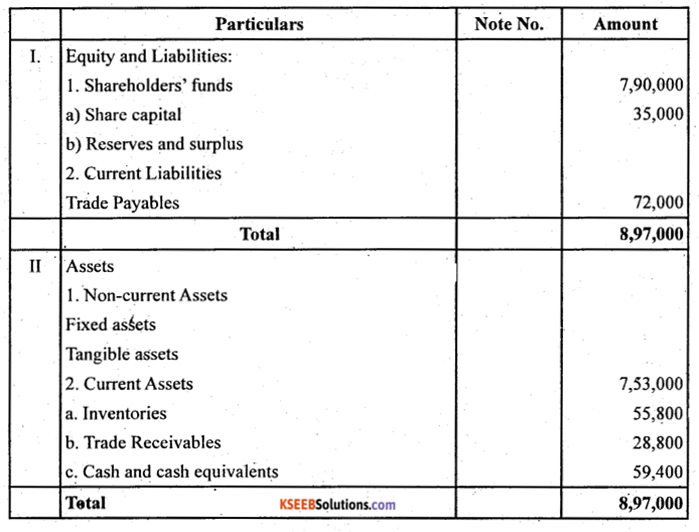Current assets = Stock + Debtors + Cash at bank
= 55,800 + 28,800 + 59,400 = 1,44,000
= 72,000
Current Ratio = $$\frac{1,44,000}{72,000}=\frac{2}{1}$$ = 2:1

Question 2.
Following is the Balance Sheet of Title Machine Ltd. as at March 31,2015.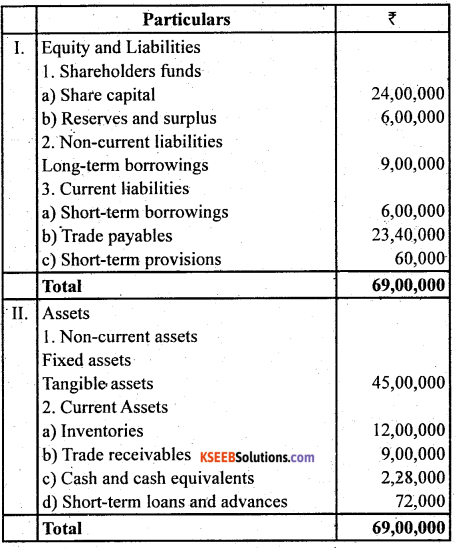Calculate Current Ratio and Liquid Ratio.Current Assets = Inventories + Trade Receivables + Cash + Short term Loans and Advances
= 12,00,000+ 9,00,000+ 2,28,000+ 60,000 = ₹ 24,00,000
Current Liabilities = Trade Payables + Short-term Borrowing + Short-term Provisions
= 23,40,000 + 6,00,000 = 60,000 = ₹ 30,00,000

2. Quick RatioQuick Assets = Trade Receivables + Cash + Short term Loans and Advances,
= 9,00,000 + 2,28,000 + 72,000 = ₹ 12,00,000

Question 3.
Current Ratio is 3.5 : 1. Working Capital is ₹ 90,000. Calculate the amount of Current Assets and Current Liabilities.or, Current Assets = 3.5 Current Liabilities (1)
Working Capital = Current Assets – Current
Liabilities Working Capital = 90,000
or, Current Assets – Current Liabi lities = 90,000
or, 3.5 = Current Liabilities – Current Liabilities = 90,000 (from I)
or, 2.5 Current Liabilities = $$\frac { 90,000 }{ 2.5 }$$ = 36,000
or Current Assets = 3.5 Current Liabilities
= 3.5 × 36,000= 1,26,000

Question 4.
Shine Limited has current 1 and quick ratio 3 : 1; if the inventor is 36,000, calculate Current Liabilities, and Current Assets.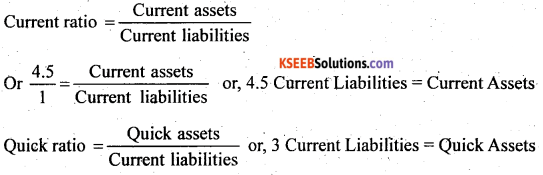Quick Assets = Current Assets – Stock
= Current Assets – 36,000
or, 4.5 Current Liabilities – 3 Current Liabilities = 36,000
or, 1.5 Current Liabilities = 36,000 or, Current Liabilities = 24,000
Current Assets = 4.5 current Liabilities
or, Current Assets = 4.5 × 24,000 = 1,08,000Question 5.
Current Liabilities of a company are ₹ 75,000. If current ratio is 4 : 1 and Liquid Ratio is 1: 1, calculate value of Current Assets, Liquid Assets and Inventory.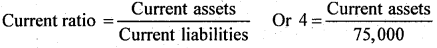or, 4 × 75,000 = Current Assets
or, Current Assets = 3,00,000Current liabilities
Liquid Assets = 75,000
Stock = Current Assets -Liquid Assets = 3,00,000 – 75,000
= 2,25,000

Question 6.
Handa Ltd. has inventory of ₹ 20,000. Total liquid assets are ₹ 1,00,000 and quick ratio is 2: 1. Calculate current ratio.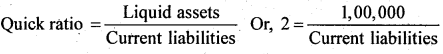Or, Current liabilities = $$\frac{1,00,000}{2}$$ = 50,000
Current Assets = Liquid Assets + inventory
= 1,00,000 + 20,000 = 1,20,000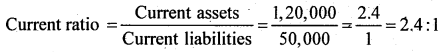Question 7.
Calculate debt-equity ratio from the following information:
Total Assets ₹ 15,00,000
Current Liabilities ₹ 6,00,000
Total Debts ₹ 12,00,000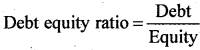Equity = Total Assets – Total Debts
= 15,00,000 – 12,00,000 = 3,00,000

Long Term Debts = Total  Debts – Currrent Liabilities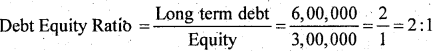Question 8.
Calculate Current Ratio if:
Inventory is ₹ 6,00,000; Liquid Assets ₹ 24,00,000; Quick Ratio 2 : 1.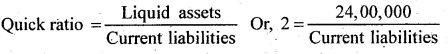Current liabilities = $$\frac { 24,00,000 }{ 2 }$$ = 12,00,000
Current Assets = Liquid Asset + Inventory = 24,00,000 + 6,00,000 = 30,00,000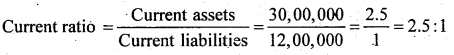Question 9.
Compute Inventory Turn over Ratio from the following information:
Net Revenue from Operations ₹ 2,00,000
Gross Profit ₹ 50,000 .
Inventory at the end ₹ 60,000
Excess of inventory at the end over
inventory in the beginning ₹ 20000Cost of Goods sold = Net Sales – Gross Profit
= 2,00,000 – 50,000= 1,50,000
Opening Stock = Closing Stock – 20,000
= 60,000 – 20,000 = 40,000Average Inventory = $$\frac{40,000+60,000}{2}=\frac{1,00,000}{2}$$ = 50,000
Stock turnover ratio = $$\frac{1,50,000}{50,000}=\frac{3}{1}$$ = 3 timesQuestion 10.
Calculate following ratios from the following information:
(i) Current ratio
(ii) Liquid ratio
(iii) Operating Ratio
(iv) Gross profit ratio
Current Assets . ₹ 7 35,000
Current Liabilities ₹ 17,500
Inventory ₹ 15,000
Operating Expenses ₹ 20,000
Revenue from Operations ₹ 60,000
Cost of Revenue from operation ₹ 30,000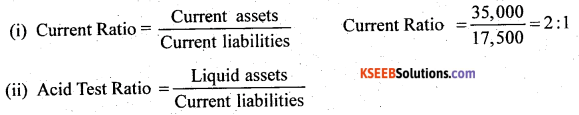Liquid assets = current assets – stock
=35,000- 15,000 = 20,000
Acid test ratio = $$\frac{20,000}{17,500}=\frac{1.413}{1}$$ = 1.143:1

Question 11.
From the following information calculate:
1. Gross Profit Ratio
2. inventory Turnover Ratio
3. Current Ratio
4. Liquid Ratio
5. Net Profit Ratio
6. Working Capital Ratio:
Revenue from Operations ₹15,20,000
Net Profit ₹ 3,60,000
Cost of Revenue from Operations ₹ 19,20,000
Long-term Debts ₹ 9,00,000
Average 7 aventory ₹ 8,00,000
Current Assets ₹ 7,60,000
Fixed Assets ₹ 14,40,000
Current Liabilities ₹ 6,00,000
Net Profit before Interest and Tax ₹ 8,00,000
1.Gross Profit = Revenue from operation – Cost of Sales
= 25,20,000- 19,20,000 = 6,00,000
Gross Profit ratio = $$\frac{6,00,000}{25,20,000} \times 100$$ = 23.81

2.$$=\frac{19,20,000}{8,00,000}=\frac{2.4}{1}=2.4: 1$$

3.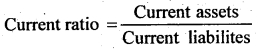Current Asset = Liquid Assets + Inventory
= 7,60, 000 + 8,00,000 = 15,60,000
Current ratio = $$\frac{15,60,000}{6,00,000}=\frac{2.6}{1}=2.6: 1$$

4.5.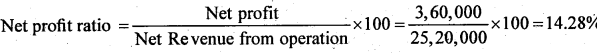6.Working capital = Current Assets – Current Liabilities
= 15,60,000 – 6,00,000 = 9,60,000
Working Capital Ratio $$=\frac{25,20,000}{9,60,000}$$ = 2.625 times .

Question 12.
Compute Gross Profit Ratio, Working Capital Turnover Ratio, Debt Equity Ratio and Proprietary Ratio from the following information:
Paid-up Share Capital ₹ 5,00,000
Current Assets ₹ 4,00,000
Revenue from Operations ₹ 10,00,000
13% Debentures ₹ 2,00,000
Current Liabilities ₹ 2,80,000
Cost of Revenue from Operations ₹ 6,00,000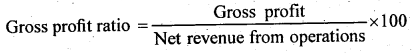Gross Profit = Net revenue from operations – Cost of Goods Sold
= 10,00,000 – 6,00,000 =4,00,000
Gross profit ratio = $$\frac{4,00,000}{10,00,000} \times 100$$ = 40%
Working Capital = Current Assets – Current Liabilities
= 4,00,000 – 2,80,000 = 1,20,000
Working Capital Ratio = $$\frac { 10,00,000 }{ 1,20,000 }$$ = 8.33 times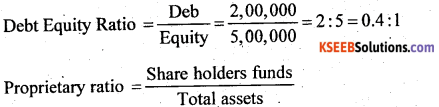Total Assets = Paid up Capital + Debentures + Current Liabilities
(∵ Total Liabilities = Total Assets)
= 5,00,000 + 2,00,000 + 2,80,000 =9,80,000
Proprietary ratio = $$\frac { 5,00,000 }{ 9,80,000 }$$ = 25:49 = 0.51:1

Question 13.
Calculate Inventory Turnover Ratio if:
Inventory in the beginning is ₹ 76,250, Inventory at the end is 98,500, Gross Revenue from Operations is ₹ 5,20,000, Sales Return is ₹ 20,000 Purchases is ₹ 3,22,250.
Ans:
Gross Profit Ratio = $$\frac { 4,00,000 }{ 10,00,000 }$$ × 100 = 40%
Cost of Goods Sold = Opening Inventory + Purchases – Closing Inventory
= 76,250 + 3,22,250 – 98,500 = 3,00,000Stock Turunover Ratio = $$\frac { 3,00,000 }{ 87,375 }$$ = 3,43 timesQuestion 14.
Calculate inventory Turnover Ratio from the data given below:
Inventory in the beginning of the year ₹ 10,000
Stock at the end of the year ₹ 5,000
Carriage ₹ 2,500
Revenue from Operations ₹ 50,0130
Purchases ₹ 25,000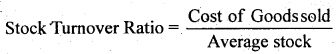Cost of Goods Sold = Opening Stock + Purchases + Carriage – Closing Stock
= 10,000 + 25,000 + 2,500-5,000 = 32,500Stock turnover ratio = $$\frac{32,500}{7,500}$$ = 4.33 times

Question 15.
A trading firm’s average inventory is ₹ 20,000 (cost). If the inventory turnover ratio is 8 times and the firm sells goods at a profit f 20% on sales, ascertain the profit of the firm.or, Cost of Goods Sold = 20,000 × 8
or, Cost of Goods Sold = 1,60,000
Let Sale Price be ₹ 100
Then Profit is ₹ 20
Hence, the Cost of Revenue from Operations = ₹ 100
₹ 20 = ₹ 80 If the Cost of Revenue from Operations is ₹ 80, then Revenue from operations = 100
If the Cost of Revenue from Operations is ₹ 1, then Revenue from operations = $$\frac { 100 }{ 80 }$$
If the Cost ofGoods Sold is 1,60,000 then Sales = $$\frac { 100 }{ 80 }$$ × 1,60,000 = 2,00,000
Profit = Revenue from Operations
=2,00,000 – 1,60,000 = ₹ 40,000

Question 16.
You are able to collect the following information about a company for two years: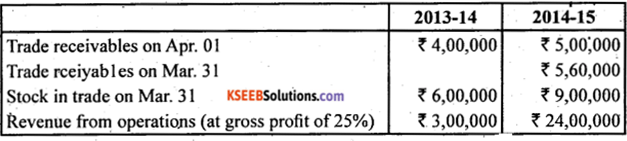Calculate Inventory Turnover Ratio and Trade Receivables Turnover Ratio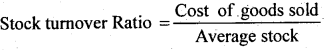or, Cost of Revenue from Operations = Revenue from operations – Gross Profit
or, Gross Profit = 25% of Sales
= 25% of 24,00,000 =6,00,000
or, Cost of Goods sold = 24,00,000 – 6,00,000 = 18,00,000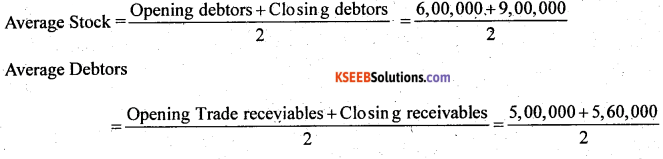Debtors Turnover Ratio = $$\frac { 24,00,000 }{ 5,30,000 }$$ = 4,53 times
Note: It has been assumed that all Revenue from operations are credit Revenue from operations

Question 17.
From the following Balance Sheet and other information, calculate following ratios:
1. Debt-Equity Ratio
2. Working Capital Turnover Ratio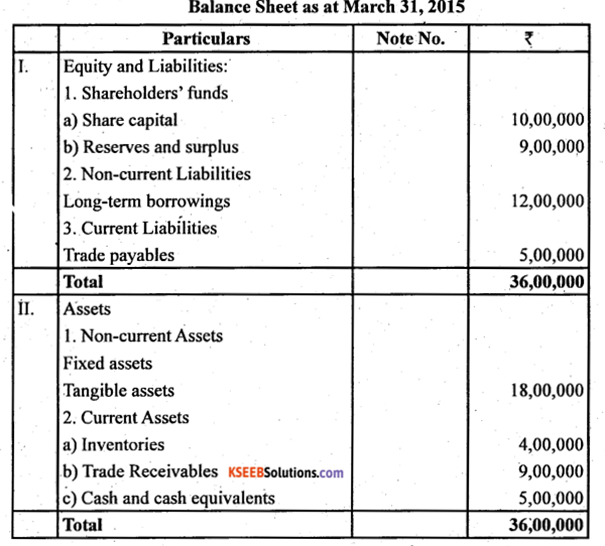Additional Information: Revenue from Operations ₹ 18,00,000 (Debt-Equity Ratio 0.63 : 1; Working Capital Turnover Ratio 1.39 times; Trade Receivables Turnover Ratio 2 times)
1. Debt – Equity RatioDebt = Long Term Borrowings = ₹ 12,00,0000
Equity = share Capital + Reserve and Surplus
= 10,00,000 + 9,00,000 = ₹ 19,00,000

2. Working Capital Turnover Ratio
Working Capital Turnover Ratio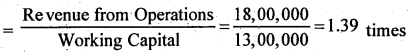Revenue from Operations = ₹ 18,00,000
Working capital = Current Assets – Current Liabilities
= 18,00,000 – 5,00,000 = ₹ 13,00,000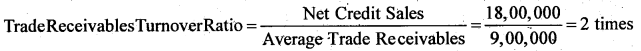Net credit sales = ₹ 18,00,000
Avereagre Trade Receivables = ₹ 9,00,000
Notes:
1. Revenue from Operations are assumed to be revenue generated from credit sales.
2. The amount of trade receivables given in the Balance Sheet is assumed to be Average Trade Receivables.Question 18.
From the following information, calculate the following ratios:
1. Liquid Ratio
2. Inventory turnover ratios
3. Return on investment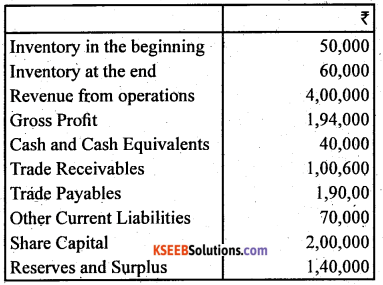1.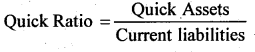Quick Assets = Cash + Debtors
= 40,000+ 1,00,000 = 1,40,000
Current Liabilities = Creditors + Outstanding Expenses
= 1,90,000 + 70,000 = 2,60,000
Quick Ratio = $$\frac{1,40,000}{2,60,000}$$ =7:13 = 0.54:1

2.Cost of Revenue from Operations = Revenue from Operations – Gross profit
= 4,00,000- 1,94,000 = 2,06,000$$=\frac{50,000+60,000}{2}=55,000$$
Inventory Turnover Ratio = $$\frac{2,06,000}{55,000}$$ = 3.74 timesCapital Employed = Equity share capital + Profit and Loss
= 2,00,000 + 1,40,000 = 3,40,000
Return on Investment = $$\frac{1,40,000}{3,40,000}$$ × 100 – 41.17%

Question 19.
From the following, calculate
(a) Debt-Equity Ratio
(b) Total Assets to Debt Ratio
(c) Proprietary Ratio.Equity / Share holders Funds = Equity Share Capital + Preference Share Capital + General Reserve +Accumulated Profit – Preliminary Expenses Written off
= 75,000 + 25,000 + 50,000 + 30,000 – 5,000=1,75,000

Question 20.
Cost of Revenue from Operations is ₹ 1,50,000. Operating expenses are ₹ 60,000. Revenue front Operations is ₹ 2,50,000. Calculate Operating Ratio.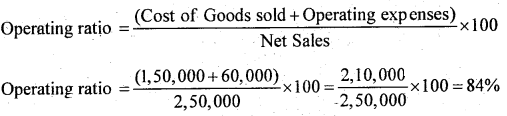Question 21.
Calculate the following ratio on the basis of following information:
1. Gross Profit Ratio
2. Current Ratio
3. Acid Test Ratio
4. Inventory Turnover Ratio
5. Fixed Assets Turnover Ratio1.2.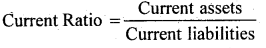Current Assets = Inventory + Trade Receivables + Cash and Cash Equivalents
= 15,000 + 27,500 + 17,500 = 60,000
Currebt Ratio = $$\frac{60,000}{40,000}=1.5: 1$$

3.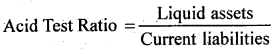Liquid Assets = Current Assets – Inventory
= 60,000 – 15,000 = 45,000
Acid test ratio = $$\frac{45,000}{40,000}=1.125: 1$$

Question 22.
From the following information calculate Gross Profit Ratio, Inventory Ratio and Trade Receivable Turnover Ratio.
Revenue from Operations ₹ 2,00,000
Cost Of Revenue from Operations ₹ 2,40,000
Inventory at the end ₹ 62,000
Gross Profit ₹ 60,000
Inventory in the beginning ₹ 58,000Gross Profit = Net Revenue from Operation – Cost of Goods Sold
= 3,00,000 – 2,40,000 = 60,000
Gross Profit Ratio $$=\frac{60,000}{3,00,000}$$ × 100 = 20%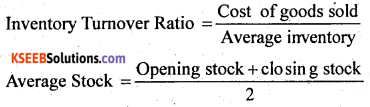$$=\frac{58,000+62,000}{2}=\frac{1,20,000}{2}=60,000$$
Stock. Turnover Ratio = $$\frac{2,40,000}{60,000}$$ = 4 times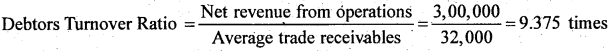Note: In the solution, the Trade receivables are assumed as the average Trade receivableserror: Content is protected !!